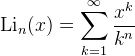Computes the polylogarithm function.

Namespace:  Meta.Numerics.Functions
Assembly:  Meta.Numerics (in Meta.Numerics.dll) Version: 4.1.4Syntax
```public static double PolyLog(
int n,
double x
)```

#### Parameters

n
Type: SystemInt32
The order, which must be non-negative.
x
Type: SystemDouble
The argument, which must be less than or equal to one.

#### Return Value

Type: Double
The value of Lin(x).Exceptions
ExceptionCondition
ArgumentOutOfRangeExceptionn is negative or x is greater than one.Remarks

The nth polylog of x is defined via the series:Its name comes from the fact that this is a generalization of the logarithm series. For n = 1 it reduces to -log(1-x). For n = 2 it reduces to the DiLog(Double) function.

The polylogarithm function becomes complex for arguments larger than one.See Also